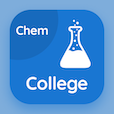Computer Science Online Courses

Digital Logic Design MCQs

Digital Logic Design MCQ PDF - Topics

# Basic Theorems and Properties of Boolean Algebra MCQ Quiz Online

Learn Basic Theorems and Properties of Boolean Algebra Multiple Choice Questions (MCQ), Basic Theorems and Properties of Boolean Algebra quiz answers PDF to study digital logic design online course for digital logic design classes. Boolean Algebra and Logic Gates Multiple Choice Questions and Answers (MCQs), Basic Theorems and Properties of Boolean Algebra quiz questions to learn free online courses. "Basic Theorems and Properties of Boolean Algebra MCQ" PDF Book: canonical and standard forms, basic definition of boolean algebra, boolean functions in dld, basic theorems and properties of boolean algebra test prep for bachelor's degree in computer science.

"According to the Boolean algebra absorption law, which of the following is correct?" MCQ PDF: basic theorems and properties of boolean algebra with choices x+xy=x, (x+y)=xy, xy+y=x, and x+y=y to learn free online courses. Study basic theorems and properties of boolean algebra quiz questions for merit scholarship test and certificate programs for computer science programs.

## MCQs on Basic Theorems and Properties of Boolean Algebra Quiz

MCQ: According to the Boolean algebra absorption law, which of the following is correct?

x+xy=x
(x+y)=xy
xy+y=x
x+y=y

MCQ: In Boolean algebra Multiplicative inverse is

0
1
1/a
a

MCQ: According to the Boolean algebra theorems x.x is equal to

x
1
0
x'

MCQ: According to Boolean algebra, postulate 2 w.r.t addition is

x+0=x
x+0=1
x+0=0
x+1=0

MCQ: According to Boolean algebra Involution law (x')' Is equal to

x'
x
1
0

### More Topics from Digital Logic Design Course